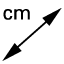# Calculating Sides in Similar Figures

Written Work - Copy into your book: We can also use simple equations to calculate matching sides in similar shapes.

## Copy the following example into your workbook

Written Work - Copy into your book: Copy the diagrams and complete the following tasks 1) Find the length of JH using equal ratios. 2) Find the length of BC using equal ratios. 3) Check your answers by using the length toolfrom the measurement menuAfter selecting the, point to and select the correct line interval. The value should appear next to the interval.
Written Work - Copy into your book: Copy the diagrams and show full working to solve the problem. Then type your answer in the space provided.

## Type the number only (without measurement units)

The value of k is:

## Type the number only (without measurement units)

The value of s is:

## Type the number only (without measurement units)

The value of x is: